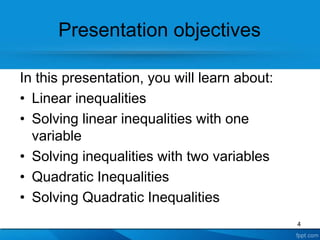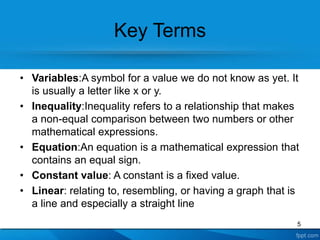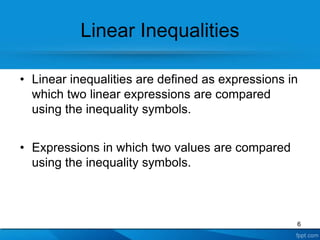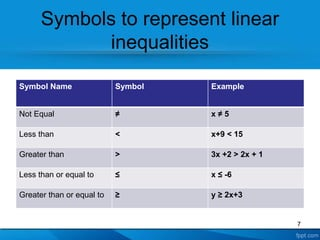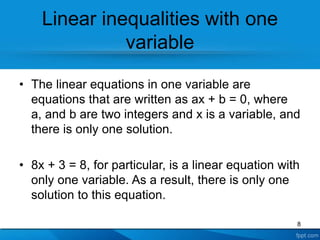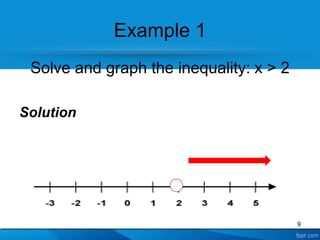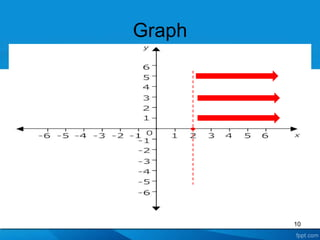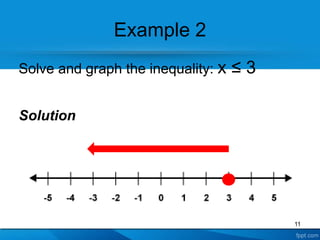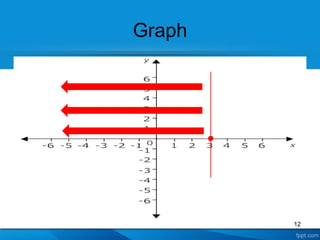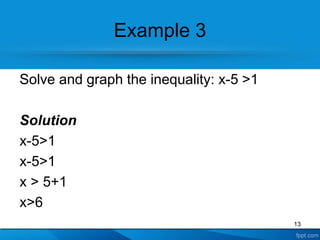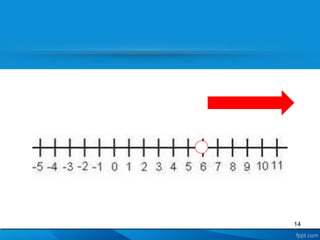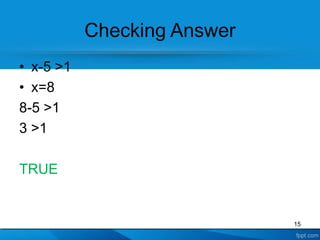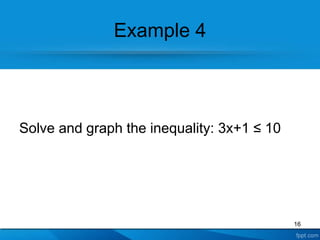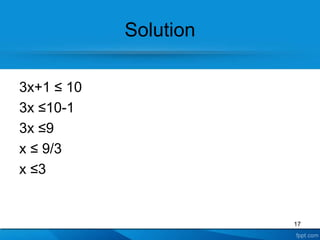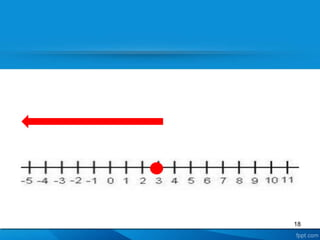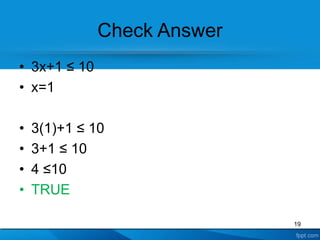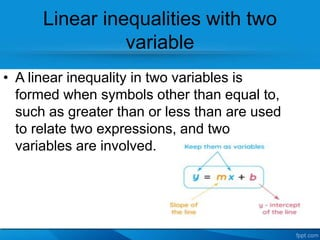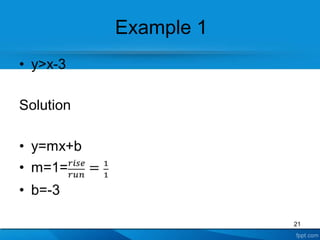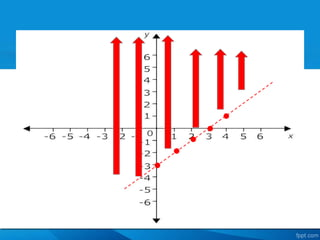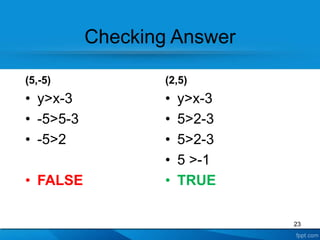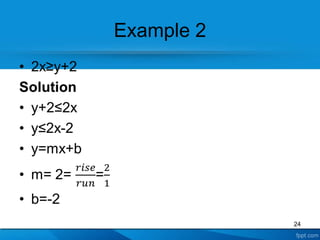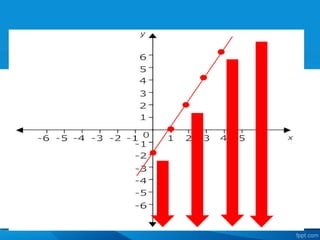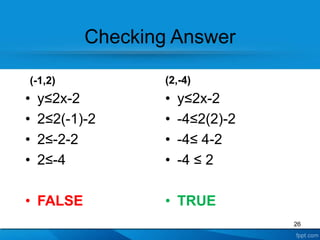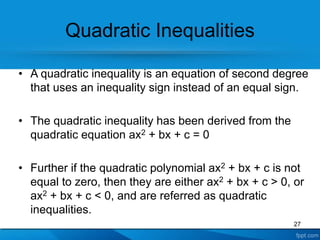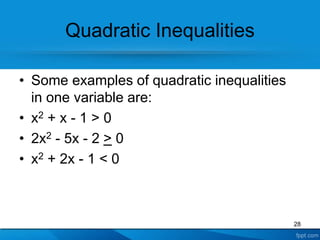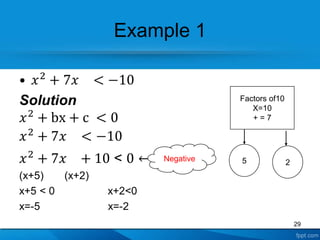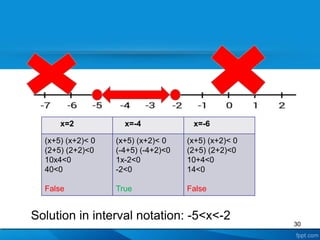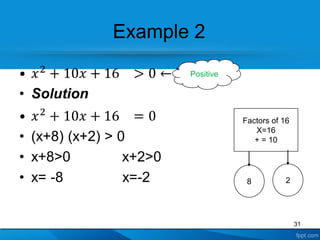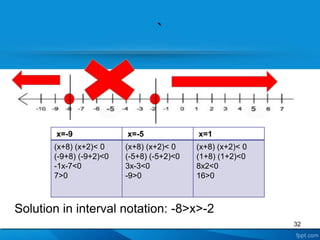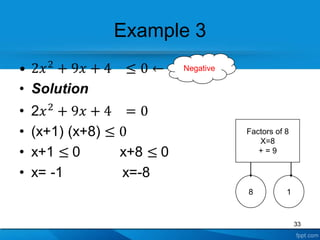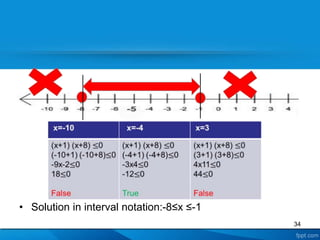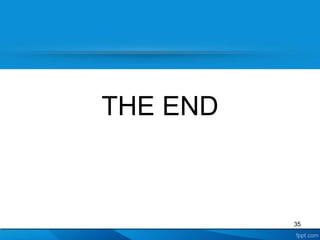1 of 35

### algebra and geometry ppt1.ppt

1. UNIVERSITY OF GUYANA EMA 3102: BASIC ALGEBRA AND GEOMETRY LECTURER: MR. PETER WINTZ 1
2. GROUP C MEMBERS • Devishwar Bahadur • Keith Damon • Tanna James • Judy Kendall • Shanaz Hosain • Ophela Johnson • Shelana Cornelius • Towana Johnson 2
3. THE SOLUTION SET OF LINEAR AND QUADRATIC INEQUALITIES 3
4. Presentation objectives In this presentation, you will learn about: • Linear inequalities • Solving linear inequalities with one variable • Solving inequalities with two variables • Quadratic Inequalities • Solving Quadratic Inequalities 4
5. Key Terms • Variables:A symbol for a value we do not know as yet. It is usually a letter like x or y. • Inequality:Inequality refers to a relationship that makes a non-equal comparison between two numbers or other mathematical expressions. • Equation:An equation is a mathematical expression that contains an equal sign. • Constant value: A constant is a fixed value. • Linear: relating to, resembling, or having a graph that is a line and especially a straight line 5
6. Linear Inequalities • Linear inequalities are defined as expressions in which two linear expressions are compared using the inequality symbols. • Expressions in which two values are compared using the inequality symbols. 6
7. Symbols to represent linear inequalities Symbol Name Symbol Example Not Equal ≠ x ≠ 5 Less than < x+9 < 15 Greater than > 3x +2 > 2x + 1 Less than or equal to ≤ x ≤ -6 Greater than or equal to ≥ y ≥ 2x+3 7
8. Linear inequalities with one variable • The linear equations in one variable are equations that are written as ax + b = 0, where a, and b are two integers and x is a variable, and there is only one solution. • 8x + 3 = 8, for particular, is a linear equation with only one variable. As a result, there is only one solution to this equation. 8
9. Example 1 Solve and graph the inequality: x > 2 Solution 9
10. Graph 10
11. Example 2 Solve and graph the inequality: x ≤ 3 Solution 11
12. Graph 12
13. Example 3 Solve and graph the inequality: x-5 >1 Solution x-5>1 x-5>1 x > 5+1 x>6 13
14. 14
15. Checking Answer • x-5 >1 • x=8 8-5 >1 3 >1 TRUE 15
16. Example 4 Solve and graph the inequality: 3x+1 ≤ 10 16
17. Solution 3x+1 ≤ 10 3x ≤10-1 3x ≤9 x ≤ 9/3 x ≤3 17
18. 18
19. Check Answer • 3x+1 ≤ 10 • x=1 • 3(1)+1 ≤ 10 • 3+1 ≤ 10 • 4 ≤10 • TRUE 19
20. Linear inequalities with two variable • A linear inequality in two variables is formed when symbols other than equal to, such as greater than or less than are used to relate two expressions, and two variables are involved. 20
21. Example 1 21
22. 22
23. Checking Answer (5,-5) • y>x-3 • -5>5-3 • -5>2 • FALSE (2,5) • y>x-3 • 5>2-3 • 5>2-3 • 5 >-1 • TRUE 23
24. Example 2 24
25. 25
26. Checking Answer (-1,2) • y≤2x-2 • 2≤2(-1)-2 • 2≤-2-2 • 2≤-4 • FALSE (2,-4) • y≤2x-2 • -4≤2(2)-2 • -4≤ 4-2 • -4 ≤ 2 • TRUE 26
27. Quadratic Inequalities • A quadratic inequality is an equation of second degree that uses an inequality sign instead of an equal sign. • The quadratic inequality has been derived from the quadratic equation ax2 + bx + c = 0 • Further if the quadratic polynomial ax2 + bx + c is not equal to zero, then they are either ax2 + bx + c > 0, or ax2 + bx + c < 0, and are referred as quadratic inequalities. 27
28. Quadratic Inequalities • Some examples of quadratic inequalities in one variable are: • x2 + x - 1 > 0 • 2x2 - 5x - 2 > 0 • x2 + 2x - 1 < 0 28
29. Example 1 29 Factors of10 X=10 + = 7 5 2 Negative
30. Solution in interval notation: -5<x<-2 30 x=2 x=-4 x=-6 (x+5) (x+2)< 0 (2+5) (2+2)<0 10x4<0 40<0 False (x+5) (x+2)< 0 (-4+5) (-4+2)<0 1x-2<0 -2<0 True (x+5) (x+2)< 0 (2+5) (2+2)<0 10+4<0 14<0 False
31. Example 2 31 Factors of 16 X=16 + = 10 8 2 Positive
32. ` 32 Solution in interval notation: -8>x>-2 x=-9 x=-5 x=1 (x+8) (x+2)< 0 (-9+8) (-9+2)<0 -1x-7<0 7>0 (x+8) (x+2)< 0 (-5+8) (-5+2)<0 3x-3<0 -9>0 (x+8) (x+2)< 0 (1+8) (1+2)<0 8x2<0 16>0
33. Example 3 33 Factors of 8 X=8 + = 9 8 1 Negative
34. • Solution in interval notation:-8≤x ≤-1 34
35. THE END 35